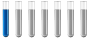## SOS with equilibrium constant when Volume changed

Chemistry and homework help forum.

Organic Chemistry, Analytical Chemistry, Biochemistry, Physical Chemistry, Computational Chemistry, Theoretical Chemistry, High School Chemistry, Colledge Chemistry and University Chemistry Forum.

Share your chemistry ideas, discuss chemical problems, ask for help with scientific chemistry questions, inspire others by your chemistry vision!

Please feel free to start a scientific chemistry discussion here!

Discuss chemistry homework problems with experts!

Ask for help with chemical questions and help others with your chemistry knowledge!

Moderators: Xen, expert, ChenBeier

johnnysuomi
NewbiePosts: 2
Joined: Thu May 12, 2022 4:06 am

### SOS with equilibrium constant when Volume changed

nitrous oxide (2 NO 2 (g) ⇌ N 2 O 4 (g) ) At 100 ° C, a 1.0 liter reaction vessel was found to contain 0.30 moles of NO 2 (g) and 0.30 moles of N 2 O 4 (g) at equilibrium.

What are the quantities of these gases when the volume of the vessel is changed to 0.50 liters and the equilibrium is restored?

(Hint: First calculate the equilibrium constant with the equilibrium concentrations and then the new equilibrium concentrations with the initial concentrations and the equilibrium constant)

Hello, This is my first post and I'm a high school student from Finland. I will really appreciate your help solving this problem
Thank you again.
ChenBeier
Distinguished MemberPosts: 1169
Joined: Wed Sep 27, 2017 7:25 am
Location: Berlin, Germany

### Re: SOS with equilibrium constant when Volume changed

1. Kc = (cNO2)^2/cN2O4
2. If Volume drop to half then pressure increases by 2 times. pV = nRT = const, what means c is also double amount.
3. Kc = (cNO2-2x)^2/(cN2O4+ x)
Solve for x